Courses

# Significant Figures Class 11 Notes | EduRev

## JEE : Significant Figures Class 11 Notes | EduRev

The document Significant Figures Class 11 Notes | EduRev is a part of the JEE Course Physics Class 11.
All you need of JEE at this link: JEE

Accuracy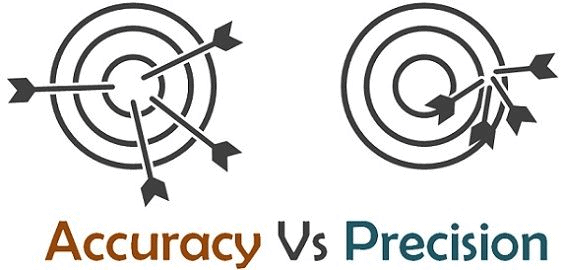• The closeness of a measured value to the actual value of the object being measured is called the accuracy of a substance. For instance, if you obtain a weight measurement of 3.2 kg for a given substance in the lab, but the actual or known weight is 10 kg, then your measurement is not accurate.
• The closeness of two or more measurements to each other is known as the precision of a substance. From the above-given example, we can figure out that, if you weigh a given substance five times, and get 3.2 kg each time, then your measurement is very precise.
• Precision is independent of accuracy. The below example will tell you about how you can be precise but not accurate and vice versa.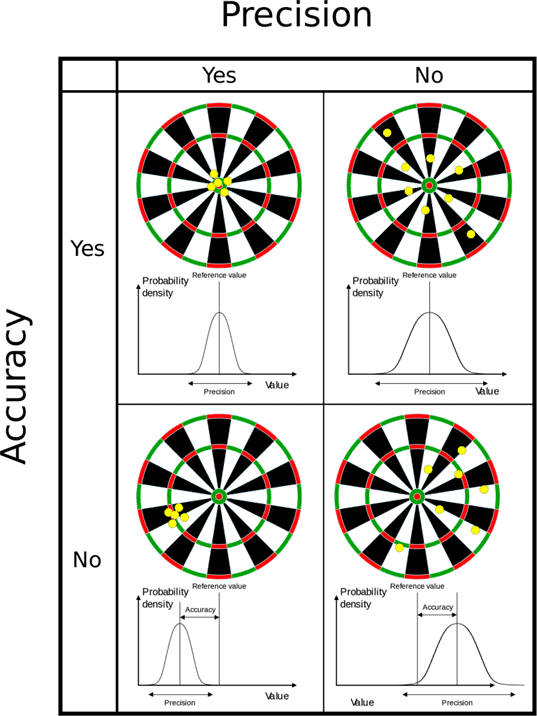Types of Precision:

• Repeatability: The variation arising when the conditions are kept identical, and repeated measurements are taken during a short time period.
• Reproducibility: The variation arising using the same measurement process among different instruments and operators, and over longer time periods.
In other words, accuracy is the degree of closeness between a measurement and the measurement’s true value.
• Precision is the degree to which repeated measurements under the same conditions are unchanged.
Try yourself:The most precise reading of the mass of an object, among the following is:
• A good analogy for understanding accuracy and precision is to imagine a football player shooting at the goal. If the player shoots into the goal, he is said to be accurate. A football player who keeps striking the same goalpost is precise but not accurate.
• Therefore a football player can be accurate without being precise if he hits the ball all over the place but still scores. A precise player will hit the ball to the same spot repeatedly, irrespective of whether he scores or not.
• A precise and accurate football player will aim at a single spot and score a goal.

Errors

The difference between the true value and the measured value of a quantity is known as the error in measurement. Errors may result from different sources and are usually classified as follows:

Systematic or Controllable Errors

Systematic errors are the errors whose causes are known. They can be either positive or negative. Due to the known causes, these errors can be minimised. Systematic errors can further be classified into three categories:

(i) Instrumental errors:-  These errors are due to imperfect design or erroneous manufacture or misuse

of the measuring instrument. These can be reduced by using more accurate instruments.

(ii) Environmental errors: These errors are due to the changes in external environmental conditions such

as temperature, pressure, humidity, dust, vibrations or magnetic and electrostatic fields.

(iii) Observational errors: These errors arise due to improper setting of the apparatus or carelessness in

taking observations.

(iv) Random Errors:

These errors are due to unknown causes. Therefore they occur irregularly and are variable in magnitude and sign. Since the causes of these errors are not known precisely, they can not be eliminated completely. For example, when the same person repeats the same observation in the same conditions, he may get different

Random errors can be reduced by repeating the observations a large number of times and taking the arithmetic

mean of all the observations. This mean value would be very close to the most accurate reading.

Note: If the number of observations is made n times then the random error reduces to (1/n) times

Example: If the random error in the arithmetic mean of 100 observations is x, then the random error in the arithmetic mean of 500 observations will be x/5

(v) Gross Errors: Gross errors arise due to human carelessness and mistakes in taking reading or calculating and recording the measurement results.
Example:
(i) Reading instrument without proper initial settings.

(ii) Taking the observations wrongly without taking necessary precautions.

(iii) Committing mistakes in recording the observations.

(iv) Putting improper values of the observations in calculations. These errors can be minimised by increasing the sincerity and alertness of the observer.

Representation of Errors

Errors can be expressed in the following ways :-

Absolute Error (Δa): The difference between the true value and the individual measured value of the quantity is called the absolute error of the measurement.

Suppose a physical quantity is measured n times and the measured values are a1, a2, a3 ..........an. The arithmetic mean (am) of these values is

am =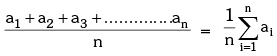If the true value of the quantity is not given then mean value (am) can be  taken as the true value. Then the absolute errors in the individual measured values are –

Δa1 = am – a1

Δa2 = am – a2

.........
Δan = am – an

The arithmetic mean of all the absolute errors is defined as the final or mean absolute error (Δa)m or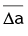of the value of the physical quantity a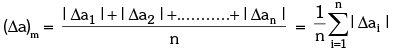So if the measured value of a quantity be 'a' and the error in measurement be Δa, then the true value (at) can be written as at = a ± Δa

Relative or Fractional  Error: It is defined as the ratio of the mean absolute error

((Δa)m or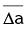) to the true value or the mean value (am or ã ) of the quantity measured.

Relative or fractional error = Mean absolute error/Mean Value = (Δa)m/aor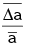When the relative error is expressed in percentage, it is known as percentage error, percentage error = relative error × 100

or percentage error = mean absolute error/true value x 100% =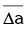/a x 100%

Propagation of Errors in Mathematical Operations

Rule I: The maximum absolute error in the sum or difference of the two quantities is equal to the sum of the absolute errors in the individual quantities.

If X = A + B or X = A – B and if ± ΔA and ± ΔB represent  the absolute errors in A and B respectively, then the maximum absolute error in X is ΔX = ΔA + ΔB   and  Maximum percentage error = ΔX/X x 100

The result will be written as  X ± ΔX (in terms of absolute error) or X ± ΔX/X 100% X (in terms of percentage error)

Rule II: The maximum fractional or relative error in the product or quotient of quantities is equal to the sum of the fractional or relative errors in the individual quantities.

If X = AB or X = A/B then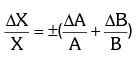Rule III: The maximum fractional error in a quantity raised to a power (n) is n times the fractional error in the quantity itself, i.e.

If X = An then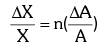If X = ApBq Cr then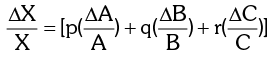If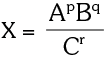then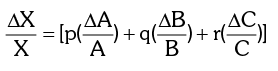Least Count

The smallest value of a physical quantity which can be measured accurately with an instrument is called its least count (L. C.).
Least Count of Vernier Callipers: Suppose the size of one main scale division (M.S.D.) is M units and that of one vernier scale division (V. S. D.) is V units. Also let the length of 'a' main scale divisions is equal to the length of 'b' vernier scale divisions.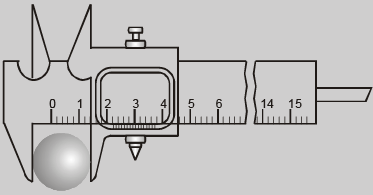The quantity (M-V) is called vernier constant (V.C) or least count (L.C) of the the vernier callipers.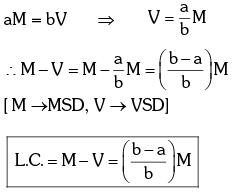Least Count of screw gauge or spherometer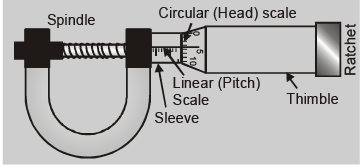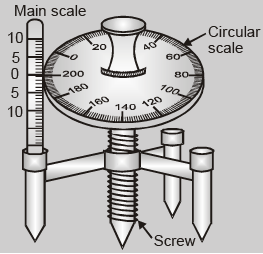Least Count = Pitch/Total no. of divisions on the circular scale

where pitch is defined as the distance moved by the screw head when the circular scale is given one complete

rotation. i.e.  Pitch = Distance moved by the screw on the linear scale/Number of full rotations given

Note: With the decrease in the least count of the measuring instrument, the accuracy of the measurement increases and the error in the measurement decreases.

The combination of Errors

Error of A Sum or A Difference

Error of A Product or A Quotient

Error in case of a measured quantity raised to a power

Error of A Sum or A Difference

Rule: When two quantities are added or subtracted, the absolute error in the final result is the sum of the absolute errors in the individual quantities.
Explanation: Let two physical quantities A and B have measured values A ± ΔA, B ± ΔB respectively, where ΔA and ΔB are their absolute errors.
We wish to find the error ΔZ in the sum
Z = A + B.

Z ± ΔZ = (A ± ΔA) + (B ± ΔB).
The maximum possible error in Z
ΔZ = ΔA + ΔB
For the difference Z = A – B, we have
Z ± Δ Z = (A ± ΔA) – (B ± ΔB)
= (A – B) ± ΔA ± ΔB
or,  ± ΔZ = ± ΔA ± ΔB
The maximum value of the error ΔZ is again ΔA + ΔB.

Error of A Product or A Quotient

Rule: When two quantities are multiplied or divided, the relative error in the result is the sum of the relative errors in the multipliers.
Explanation: Let Z = AB and the measured values of A and B are A ± ΔA and B ± ΔB. Then
Z ± ΔZ = (A ± ΔA) (B ± ΔB)
Z ± ΔZ = AB ± B ΔA ± A ΔB ± ΔA ΔB
Dividing LHS by Z and RHS by AB we have,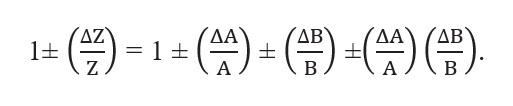Since ΔA and ΔB are small, we shall ignore their product.
Hence the maximum relative error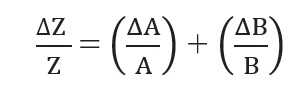You can easily verify that this is true for division also.

Error in case of a measured quantity raised to a power

Rule: The relative error in a physical quantity raised to the power k is the k times the relative error in the individual quantity.
Explanation: Suppose Z = A2,
Then,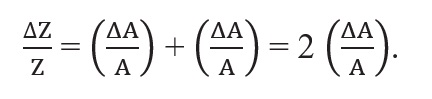Hence, the relative error in Ais two times the error in A.
In general, if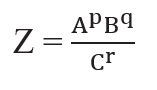Then,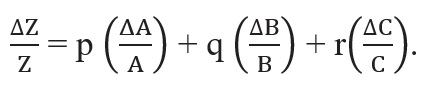The physical quantity Z with absolute error ∆Z is then expressed as, Z ± ∆Z

Offer running on EduRev: Apply code STAYHOME200 to get INR 200 off on our premium plan EduRev Infinity!

## Physics Class 11

75 videos|312 docs|152 tests

,

,

,

,

,

,

,

,

,

,

,

,

,

,

,

,

,

,

,

,

,

;